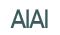# A Minimal Ontology for OBO and GO

A minimal OWL-Full ontology for Open Biological Ontology (OBO) and Gene Ontology (GO) terms is proposed: http://www.aiai.ed.ac.uk/resources/go/obo.owl defining the namespace obowl.
This ontology defines the vocabulary that might be used in the various anatomies/ontologies, and not the domain-level content. The ontology is in OWL Full as it defines relations that hold of classes (e.g. partOf) as is the case in GO. The existing OBO ontologies lack necessary and sufficient information to create OWL Description Logic definitions - hence stepping up a complexity level to OWL Full is required (in the short/medium term).

For a Ontology Editor that exports/imports this format see COBrA or download COBrA.

### Mapping GO to OWL Full

GO terms and relations are defined in a RDF/XML syntax that does not make any use of an explicit ontology, or the RDF Schema vocabulary. These elements need to be mapped to OWL Full terms, and the technique is as follows.

GO terms are mapped to Classes in RDFS/OWL, either as instances of obowl:ObjectClass, or as subclasses instances of obowl:EventClass. While GO/OBO terms are sometimes better modelled as instances, they are intended to have a 'class' or 'type' level coverage, hence the mapping to classes is appropriate. The distinction between Objects and Events is a simple, standard way to distinguish concrete, or even abstract, things that endure through time, from things that 'happen'. Much more detail could to be added: TimePoints etc but we do not do that (yet) as such concepts are not necessary to define the relations we are most interested in: isa (subClassOf) and part-of.

The GO is_a relation is directly replaced with rdfs:subClassOf. Note that although GO uses RDF syntax, only the URIref mechanism is used, the GO is_a relation has no defined semantics. rdfs:subClassOf has the usual set theory semantics.

The Object / Event / ObjectClass / EventClass distinctions are used to differentiate between a number of part-of relations:
isPartOf is the conventional (part-of Part Whole) relation.
partOf relates a Part-Class to a Whole-Class, i.e. it is a class-level relation. This relation is equivalent to part_of in GO, in the case where it applies to object-types, and may have one of two qualifications:
classDefinition which states that all instances of the Whole-Class have some instance of the Part-Class as a part, or
termDefinition which blocks the inference that sub-types of the Whole-Class have Part-Class as parts.

isSubEventOf is the part-of relation as applied to Events.
subEventOf relates a SubEvent-Class to a Event-Class, i.e. it is a class-level relation. This relation is equivalent to part_of in GO, in the case of processes when it applies to event-types, and has the same qualifications as partOf. Other qualifiers include:
directPartDefinition which states that no other part is intermediate between the part and the whole. This relation strengthens the part-of relation, and allows direct sub-parts to be distinguished from parts that are sub-sub-parts.
partDefinition states that the part exists only as part of the whole, hence this can be regarded as the definition of the part.

### Semantics

The following Otter axioms define the interpretation of partOf:
- partOf as a classDefinition
(all P W ((partOf(P,W) & classDefinition(P,W))
<-> (all w (exists p (type(w,W) -> (type(p,P) & isProperPartOf(p,w))))))).

- partOf as a termDefinition
(all P W ((partOf(P,W) & termDefinition(P,W))
<->
(all w (exists p ((-(exists C ((subClassOf(C,W) & type(w,C)))) &
type(w,W)) -> ( -(exists D ((subClassOf(D,P) & type(p,D)))) & type(p,P) & isProperPartOf(p,w))))))).

- direct parts
(all P W ((partOf(P,W) & directPartDefinition(P,W))
<-> (all w p ((type(w,W) & type(p,P) & isProperPartOf(p,w))
-> -(exists z (isProperPartOf(p,z) & isProperPartOf(z,w))))))).

- axioms for isPart0f and isProperPartOf
(all x y z ((isPart0f(x,y) & isPart0f(y,z)) -> isPart0f(x,z))).
(all x isPart0f(x,x)).
(all x y ((isPart0f(x,y) & isPart0f(y,x)) -> =(x,y))).
(all x y (isProperPartOf(x,y) <-> (isPart0f(x,y) & -(isPart0f(y,x))))).

- type and subClassOf
(all x y z ((type(x,y) & subClassOf(y,z)) -> type(x,z))).

Last change: Thu Nov 20 18:24:17 GMT 2003

 Dr Stuart Aitken Artificial Intelligence Applications Institute The University of Edinburgh Email: s.aitken@ed.ac.uk